#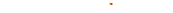###### Rotate

Basics

Rotate Type

 Constant Set fixed angle Newton Dynamics Rotate with speed and acceleration relative to the axes. Curve Value Set the fixed angle for keyframes in Timeline. Curve Speed Set the rotational speed for keyframes in Timeline.

Constant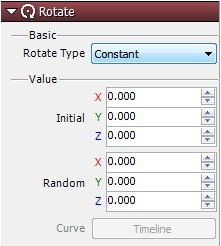Fix the initial angle so it doesn’t change. Compared to other function types, one charactersitic of fixing the initial angle is it creates a light processing load on the CPU.

Newton Dynamics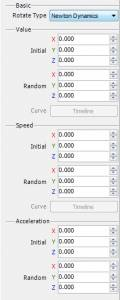Set the rotational speed and accleration for each axis. Random ranges can be set for each axis.
 Value Specify the initial positions of particles. The random value is the random range for the initial position. The random range is between -(specified value) and +(specified value). Speed Specify the rotational speeds of particles. The random value is the range for loss of rotational speed. The random range is between -(specified value) and +(specified value). Acceleration Specify the rotational acceleration of particles. The random value is the range for loss of rotational acceleration. The random range is between -(specified value) and +(specified value).

Curve Value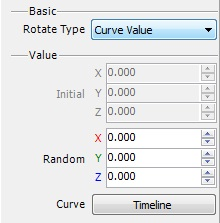Set the rotation of a fixed location for graphs in Timeline. Change keyframes in real time as they run.

Curve Speed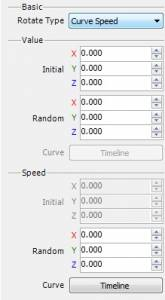Set rotational speeds for graphs in Timeline. Change keyframes in real time as they run. Set initial angles.